Stick Problem A Stick resting on ice is

• Slides: 10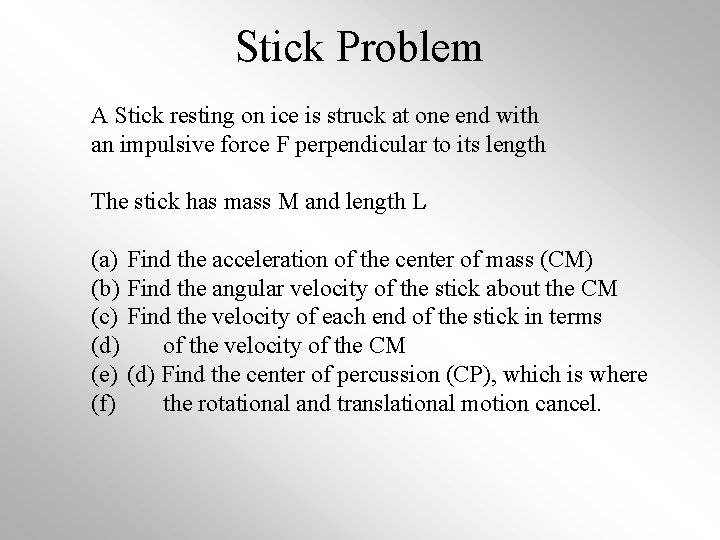Stick Problem A Stick resting on ice is struck at one end with an impulsive force F perpendicular to its length The stick has mass M and length L (a) (b) (c) (d) (e) (f) Find the acceleration of the center of mass (CM) Find the angular velocity of the stick about the CM Find the velocity of each end of the stick in terms of the velocity of the CM (d) Find the center of percussion (CP), which is where the rotational and translational motion cancel.Diagram .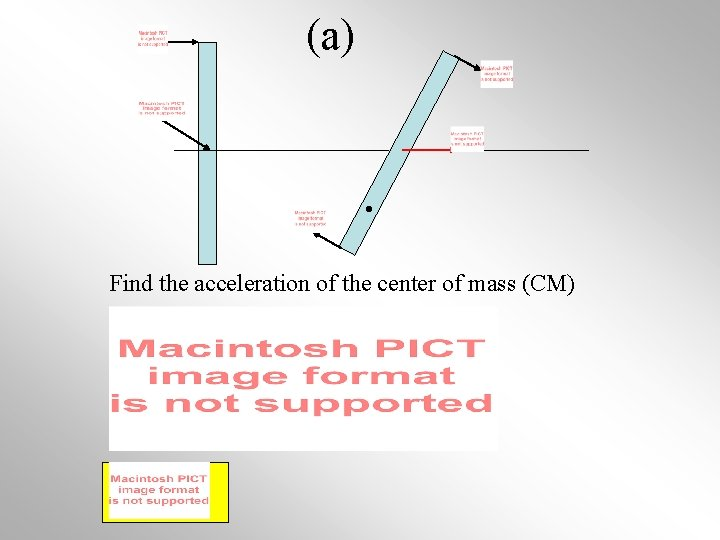(a) . Find the acceleration of the center of mass (CM)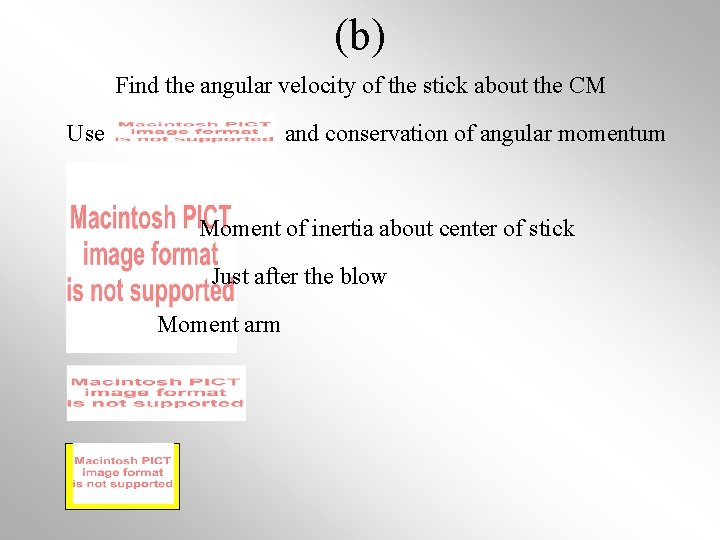(b) Find the angular velocity of the stick about the CM Use and conservation of angular momentum Moment of inertia about center of stick Just after the blow Moment arm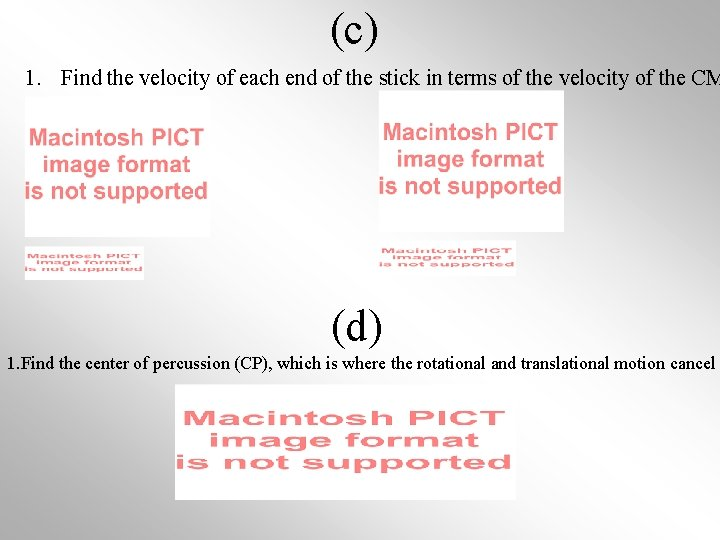(c) 1. Find the velocity of each end of the stick in terms of the velocity of the CM (d) 1. Find the center of percussion (CP), which is where the rotational and translational motion cancel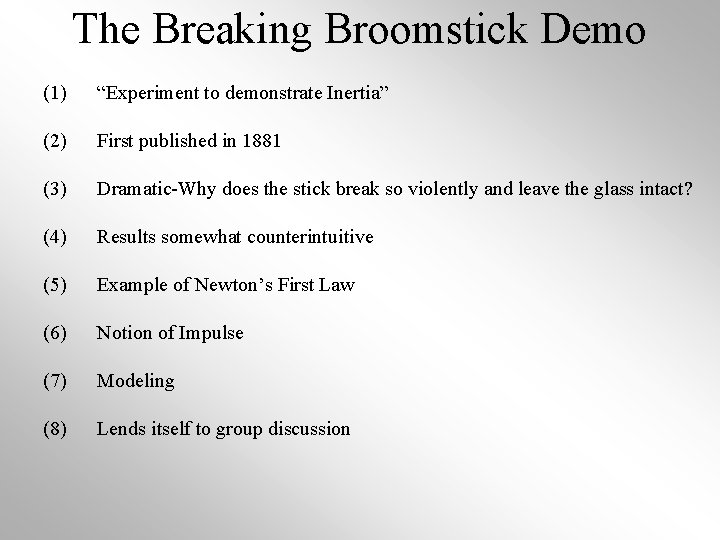The Breaking Broomstick Demo (1) “Experiment to demonstrate Inertia” (2) First published in 1881 (3) Dramatic-Why does the stick break so violently and leave the glass intact? (4) Results somewhat counterintuitive (5) Example of Newton’s First Law (6) Notion of Impulse (7) Modeling (8) Lends itself to group discussionPhysics Points (1) Motion of each half behaves similarly to a single rod receiving A blow at one end. Observe end of rod. What happens? (2) (3) Center of mass almost falls in a straight line under gravity. (4) Observe point on stick 2/3 from CM where the rotational Motion is canceled by the vertical motion. (Instantaneous axis of rotation) (5) Slight sideways constant velocity due to blow (6) Measure (7) Estimate and compare to simple model and deduce F.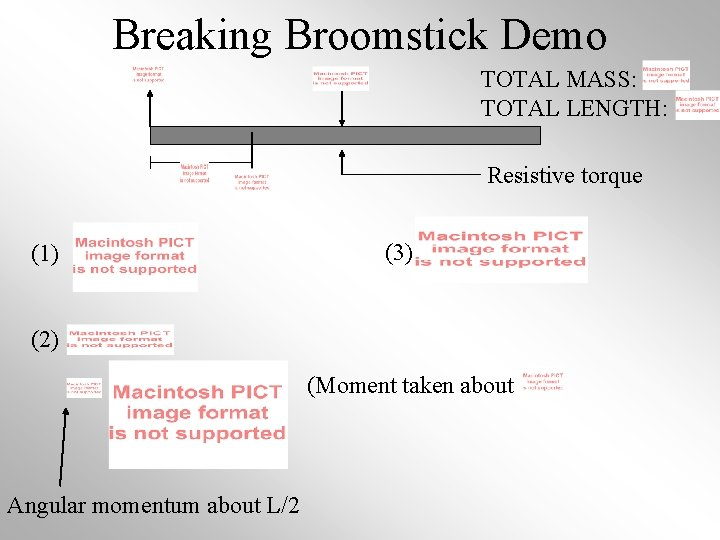Breaking Broomstick Demo TOTAL MASS: TOTAL LENGTH: Resistive torque (1) (3) (2) (Moment taken about Angular momentum about L/2Broomstick Breaking Cont. Resistive torque RIGHT SIDE LEFT SIDE w/out resistive torque w/ resistive torqueApparatus -4 ft long, 7/8 in diameter white pine, cedar, or hickory dowel rod or broomstick -Stick pins in each end; cut off heads -Support each pin with a wine glass, coke can, block of wood, etc. Need clearance -Striking stick: Steel 1/2” in diameter and 2 ft long -Mark the halfway point of stick so you know where to strike it -Use a hacksaw to etch it around the circumference; avoid stick fracturing due to other weakness. -Raise striking stick and hit the center as hard as you can; follow through